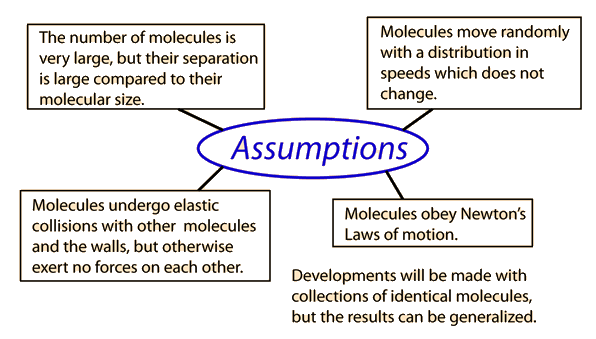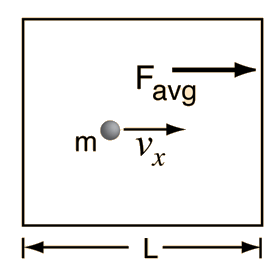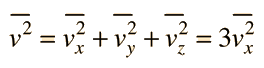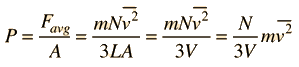# Kinetic Theory

The kinetic theory of gases is the study of the microscopic behavior of molecules and the interactions which lead to macroscopic relationships like the ideal gas law.The study of the molecules of a gas is a good example of a physical situation where statistical methods give precise and dependable results for macroscopic manifestations of microscopic phenomena. For example, the pressure, volume and temperature calculations from the ideal gas law are very precise. The average energy associated with the molecular motion has its foundation in the Boltzmann distribution, a statistical distribution function. Yet the temperature and energy of a gas can be measured precisely.

 Comment on statistical methods Comment on the role of the Boltzmann distribution
 Molecular speed distribution Separation of molecules in ideal gas
Index

Kinetic theory concepts

 HyperPhysics***** Thermodynamics R Nave
Go Back

# Newton's Laws and Collisions

Applying Newton's Laws to an ideal gas under the assumptions of kinetic theory allows the determination of the average force on container walls. This treatment assumes that the collisions with the walls are perfectly elastic.In this development, an overbar indicates an average quantity. In the expression for the average force from N molecules, it is important to note that it is the average of the square of the velocity which is used, and that this is distinctly different from the square of the average velocity.

 Determination of average pressure
 Can you weigh the gas in a closed container?
Index

Kinetic theory concepts

 HyperPhysics***** Thermodynamics R Nave
Go Back

# Gas Pressure from Kinetic Theory

Under the assumptions of kinetic theory, the average force on container walls has been determined to beThe average force and pressure on a given wall depends only upon the components of velocity toward that wall. But it can be expressed in terms of the average of the entire translational kinetic energy using the assumption that the molecular motion is random.and assuming random speeds in all directionsThen the pressure in a container can be expressed asExpressed in terms of average molecular kinetic energy:This leads to a concept of kinetic temperature and to the ideal gas law.

### Ideal gas law

Index

Kinetic theory concepts

 HyperPhysics***** Thermodynamics R Nave
Go Back